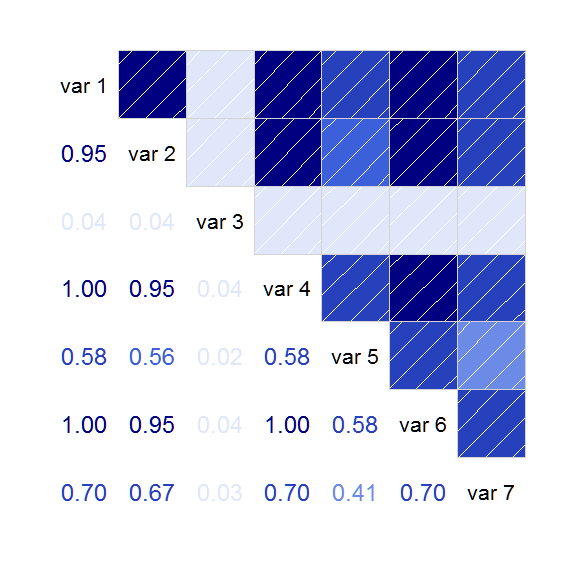# Finding the nearest proper correlation matrix

#### 2018-07-09

Consider the following matrix, as might arise from calculating covariance based on pairwise-complete data.

vv <- matrix(c(100.511, 159.266, 3.888, 59.964, 37.231, 32.944, 68.845,
159.266, 277.723, 6.161, 95.017, 58.995, 52.203, 109.09, 3.888,
6.161, 99.831, 2.32, 1.44, 1.274, 2.663, 59.964, 95.017, 2.32,
35.774, 22.212, 19.655, 41.073, 37.231, 58.995, 1.44, 22.212,
40.432, 12.203, 25.502, 32.944, 52.203, 1.274, 19.655, 12.203,
10.798, 22.566, 68.845, 109.09, 2.663, 41.073, 25.502, 22.566,
96.217), nrow=7, byrow=TRUE)
print(vv)
##         [,1]    [,2]   [,3]   [,4]   [,5]   [,6]    [,7]
## [1,] 100.511 159.266  3.888 59.964 37.231 32.944  68.845
## [2,] 159.266 277.723  6.161 95.017 58.995 52.203 109.090
## [3,]   3.888   6.161 99.831  2.320  1.440  1.274   2.663
## [4,]  59.964  95.017  2.320 35.774 22.212 19.655  41.073
## [5,]  37.231  58.995  1.440 22.212 40.432 12.203  25.502
## [6,]  32.944  52.203  1.274 19.655 12.203 10.798  22.566
## [7,]  68.845 109.090  2.663 41.073 25.502 22.566  96.217

This is not a proper covariance matrix (this one has a negative eigenvalue).

If we attempt to use the cov2cor() function to convert the covariance matrix to a correlation matrix, we find the largest correlation values are slightly larger than 1.0.

If this is passed to the corrgram function, it will issue a warning that the input data is not a correlation matrix and then calculate pairwise correlations of the columns, resulting in a non-sensical graph.

cc <- cov2cor(vv)
max(cc) # 1.000041
##  1.000041

There are several packages with functions that can be used to force the correlation matrix to be an actual, positive-definite correlation matrix. Two are given here. First, using the psych package.

require(psych)
## Loading required package: psych
## Warning: package 'psych' was built under R version 3.5.1
cc2 <- cor.smooth(cc)
## Warning in cor.smooth(cc): Matrix was not positive definite, smoothing was
## done
max(cc2)
##  1

Next, with the slighly more tricky sfsmisc package.

library(sfsmisc)
# nearcor uses 'identical' and says the matrix is not symmetric
isSymmetric(cc) # TRUE
##  TRUE
identical(cc, t(cc)) # FALSE
##  FALSE
# round slightly to make it symmetric
cc3 <- nearcor(round(cc,12))\$cor
max(cc3)
##  1

After converting the matrix to a valid correlation matrix, an accurate corrgram can be created

require(corrgram)
## Loading required package: corrgram
corrgram(cc2, lower=panel.cor)